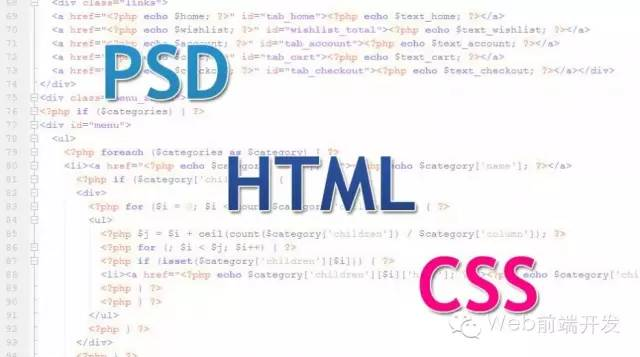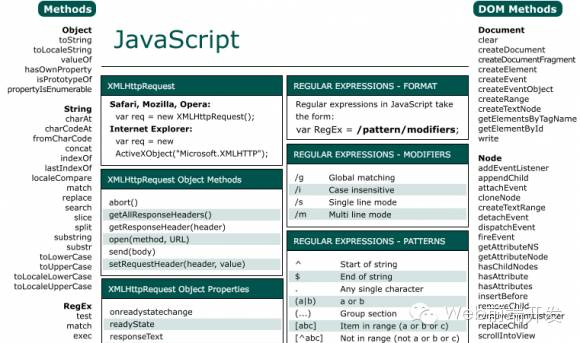#WEB前端开发成长指南

0
0
01. 云栖社区>
2. 博客>
3. 正文

## WEB前端开发成长指南

rayner 2015-09-05 22:34:00 浏览895

【背景】

web 前端研发工程师，在国内是一个朝阳职业，自07-08年正式有这个职业以来，也不过三四年的时间。这个领域没有学校的正规教育，没有行内成体系的理论指 引，几乎所有从事这个职业的人都是靠自己自学成才。自学成才，一条艰辛的坎坷路，我也是这样一路走来。从2002年开始接触web前端研发至今已然有了9 个年头，如今再回首，期间的走了很多弯路。推已及人，如果能让那些后来者少走些弯路，辛甚辛甚！【前言】

 1 2 3 4 5 `var` `str=``"www.baidu.com/?page"``;` `str=str.replace(``'?page'``,``""``);` `alert(str);` `str=str.substring(0,str.indexof(``"/"``));` `alert(str);`

 01 02 03 04 05 06 07 08 09 10 11 12 13 14 15 16 17 18 19 20 21 22 23 24 25 26 27 28 29 30 31 32 33 34 35 36 37 38 39 40 41 42 43 44 45 46 `// 计算系统当前是星期几` `var` `str = ``""``;` `var` `week = ``new` `date().getday();` `if` `(week == 0) {` `str = ``"今天是星期日"``;` `} ``else` `if` `(week == 1) {` `str = ``"今天是星期一"``;` `} ``else` `if` `(week == 2) {` `str = ``"今天是星期二"``;` `} ``else` `if` `(week == 3) {` `str = ``"今天是星期三"``;` `} ``else` `if` `(week == 4) {` `str = ``"今天是星期四"``;` `} ``else` `if` `(week == 5) {` `str = ``"今天是星期五"``;` `} ``else` `if` `(week == 6) {` `str = ``"今天是星期六"``;` `}` `// 或者更好一些` `var` `str1 = ``"今天是星期"``;` `var` `week = ``new` `date().getday();` `switch` `(week) {` `case` `0 :` `str1 += ``"日"``;` `break``;` `case` `1 :` `str1 += ``"一"``;` `break``;` `case` `2 :` `str1 += ``"二"``;` `break``;` `case` `3 :` `str1 += ``"三"``;` `break``;` `case` `4 :` `str1 += ``"四"``;` `break``;` `case` `5 :` `str1 += ``"五"``;` `break``;` `case` `6 :` `str1 += ``"六"``;` `break``;` `}` `alert(str);` `alert(str1);`1 2 3 `var` `str=``"www.baidu.com/?page"``;` `str=str.replace(/?page/,``""``);` `alert(str);`

 1 2 3 4 `var` `a = ``new` `array(``"日"``, ``"一"``, ``"二"``, ``"三"``, ``"四"``, ``"五"``, ``"六"``);` `var` `week = ``new` `date().getday();` `var` `str = ``"今天是星期"``+ a[week];` `alert(str);`1 2 3 4 5 6 7 `var` `str=``"www.baidu.com/?page"``;` `// 1、字符串剪裁` `str.substring(0, str.indexof(``"?page"``));` `// 2、正则表达式` `str.replace(/?page/, ``""``);` `// 3、字符串分拆、合并` `str.split(``"?page"``).join(``""``);`

“入室”阶段，程序员应该能够肯定的回答：对于这个需求而言，我的代码就是最优秀的代码。

 1 2 `// 计算系统当前是星期几` `var` `str = ``"今天是星期"` `+ ``"日一二三四五六"``.charat(``new` `date().getday());`

 01 02 03 04 05 06 07 08 09 10 11 12 13 14 15 16 17 18 19 20 21 `var` `str = ``"http://www.xxx.com/?pn=0"``; // 删除指定字符 pn=0` `// 我将这个字符串里所可能想到的各种情况都列举出来` `var` `a = [` `"http://www.xxx.com/vmpn=?pn=0"``// pn= 可能出现在 ? 前` `, ``"http://www.xxx.com/vmpn=?pn="``// url里允许pn 值为空` `, ``"http://www.xxx.com/vmpn=?pn=0&a=1"``// url 里可有多个字段` `, ``"http://www.xxx.com/vmpn=?a=1&pn=0"``// 可能排在最后` `, ``"http://www.xxx.com/vmpn=?a=1&pn=0&pn=1"``// 可能有多个 pn 字段` `, ``"http://www.xxx.com/vmpn=?a=1&pn=0&b=2"``// 可能在中间` `, ``"http://www.xxx.com/vmpn=?a=1&pn=0&pn=1&b=1"` `// 可能在中间成组` `, ``"http://www.xxx.com/vmpn=?a=1&pn=0&b=1&pn=1"` `// 可能零星分布` `];` `/* 需求的不言之秘：` `? 若出现在字符串最尾则要去之` `? & 两个符号不可重叠` `*/` `var` `reg = /((\?)(pn=[^&]*&)+(?!pn=))|(((\?|&)pn=[^&]*)+\$)|(&pn=[^&]*)/g;` `for` `(``var` `i = 0; i < a.length; i++) {` `alert(a + ``"\n"` `+ a.replace(reg, ``"\$2"``));` `}`01 02 03 04 05 06 07 08 09 10 11 12 13 14 15 16 17 18 19 20 21 22 23 24 25 26 27 28 29 `/**` `* 在拼接正则表达式字符串时，消除原字符串中特殊字符对正则表达式的干扰` `* @author:meizz` `* @version: 2010/12/16` `* @param {string} str 被正则表达式字符串保护编码的字符串` `* @return {string} 被保护处理过后的字符串` `*/` `function` `escapereg(str) {` `return` `str.replace(``new` `regexp(``"([.*+?^=!:\x24{}()|[\\]\/\\\\])"``, ``"g"``), ``"\\\x241"``);` `}` `/**` `* 删除url字符串中指定的 query` `* @author:meizz` `* @version:2010/12/16` `* @param {string} url url字符串` `* @param {string} key 被删除的query名` `* @return {string} 被删除指定 query 后的url字符串` `*/` `function` `delurlquery(url, key) {` `key = escapereg(key);` `var` `reg = ``new` `regexp(``"((\\?)("``+ key +``"=[^&]*&)+(?!"``+ key +` `"=))|(((\\?|&)"``+ key +``"=[^&]*)+\$)|(&"``+ key +``"=[^&]*)"``, ``"g"``);` `return` `url.replace(reg, ``"\x241"``)` `}` `// 应用实例` `var` `str = ``"http://www.xxx.com/?pn=0"``; // 删除指定字符 pn=0` `delurlquery(str, ``"pn"``);`

 01 02 03 04 05 06 07 08 09 10 11 12 13 14 15 16 17 18 19 20 21 22 23 24 25 26 27 28 29 30 31 32 33 `// 库文件 /mz/string/escapereg.js` `/**` `* 在拼接正则表达式字符串时，消除原字符串中特殊字符对正则表达式的干扰` `* @author:meizz` `* @version: 2010/12/16` `* @param {string} str 被正则表达式字符串保护编码的字符串` `* @return {string} 被保护处理过后的字符串` `*/` `mz.string.escapereg = ``function` `(str) {` `return` `str.replace(``new` `regexp(``"([.*+?^=!:\x24{}()|[\\]\/\\\\])"``, ``"g"``), ``"\\\x241"``);` `}` `// 库文件 /mz/url/delquery.js` `/// include mz.string.escapereg;` `/**` `* 删除url字符串中指定的 query` `* @author:meizz` `* @version:2010/12/16` `* @param {string} url url字符串` `* @param {string} key 被删除的query名` `* @return {string} 被删除指定 query 后的url字符串` `*/` `mz.url.delquery = ``function` `(url, key) {` `key = mz.string.escapereg(key);` `var` `reg = ``new` `regexp(``"((\\?)("``+ key +``"=[^&]*&)+(?!"``+ key +` `"=))|(((\\?|&)"``+ key +``"=[^&]*)+\$)|(&"``+ key +``"=[^&]*)"``, ``"g"``);` `return` `url.replace(reg, ``"\x241"``)` `}` `// 应用实例` `/// include mz.url.delquery;` `var` `str = ``"http://www.xxx.com/?pn=0"``; // 删除指定字符 pn=0` `mz.url.delquery(str, ``"pn"``);`

 01 02 03 04 05 06 07 08 09 10 11 12 13 14 15 16 17 18 19 20 21 `// 库文件 /mz/url/delquery.js` `/// include mz.string.escapereg;` `/**` `* 删除url字符串中指定的 query` `* @author:meizz` `* @version:2010/12/16` `* @param {string} url url字符串` `* @param {string} key 被删除的query名` `* @return {string} 被删除指定 query 后的url字符串` `*/` `string.prototype.delquery = ``function` `( key) {` `key = mz.string.escapereg(key);` `var` `reg = ``new` `regexp(``"((\\?)("``+ key +``"=[^&]*&)+(?!"``+ key +` `"=))|(((\\?|&)"``+ key +``"=[^&]*)+\$)|(&"``+ key +``"=[^&]*)"``, ``"g"``);` `return` `this``.replace(reg, ``"\x241"``)` `}` `// 应用实例` `/// include mz.url.delquery;` `var` `str = ``"http://www.xxx.com/?pn=0"``; // 删除指定字符 pn=0` `str.delquery(``"pn"``);`rayner
+ 关注